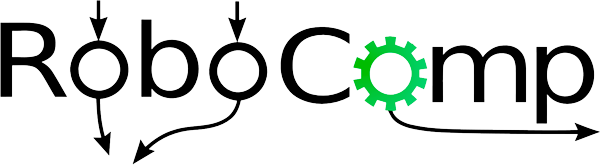# Face Recognition

26th July, 2019

In this post I will discuss our Face Recognition algorithm and its implementation. Deep Neural Networks is the current state of the art for the task of image classification and face recognition (source), but it requires large amount of labelled data and fixed number of classes. Since both of these traits are not suitable with our problem, thus we cannot train a Face Recognition model from scratch.

## Solution

Instead of directly classifying images in labels, we represent each image as a 512 dimensional feature which is a representation of mapping from face images to a compact space. The embedding is obtained from a state of the art face recognition pretrained model which can handle faces with varying viewpoints, pose and lighting conditions. This embedding space can now be modelled such that the distance between two embeddings defined by some function f is a measure of how similar two faces are.

So now, the recognition problem is converted to a clustering problem where similar faces should be clustered together based on their similarity distance. This problem is solved using a k-NN classifier with a threshold (hyperparameter) to ensure that unknown faces are not identified.

## Pretrained Model

I am using FaceNet:A Unified Embedding for Face Recognition and Clustering model implemented by David Sandberg which is trained on CASIA WebFace dataset. This model has an accuracy on 99.65% on the LFW dataset. Following are the two functions which I am currently using to measure them distance between the given neural embeddings.

### L2 Euclidean Distance

Given an embedding x1 and x2 then the distance between them is represented by the Euclidean Distance between their feature representations. If the distance is less than 1, then images are similar otherwise different.

Another possible distance representation can be a summation of product of neural embeddings elementwise. In this case if the distance lies between 0.45-0.55 then the images can be classified as belonging to the same label.

I have created a small dataset from the available Face Recognition Dataset for evaluating my model. These datasets include CACD celebrity dataset, LFW and CASIA-WebFace evaluation set. The model performs reasonably well on these datasets, but as the number of images reduces to 1-2 per class then the accuracy of the model reduces. So currently the model requires around 4-5 images for each label to recognize faces effectively.